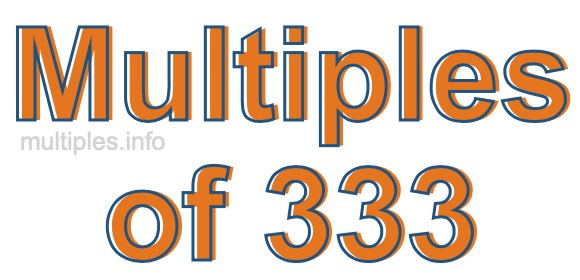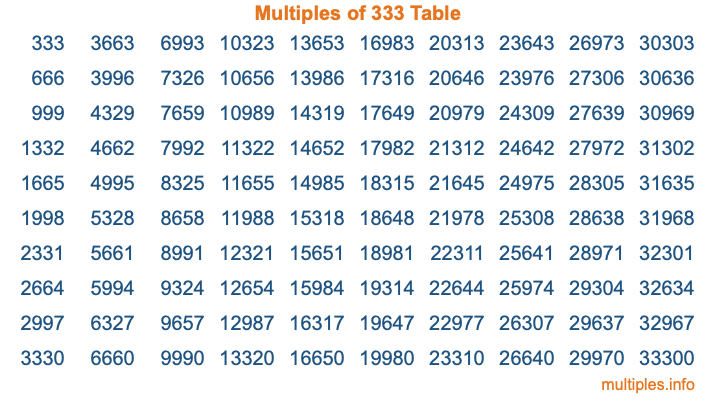Multiples of 333Welcome to the Multiples of 333 page. Here we will first teach you everything you will ever need to know about the multiples of 333, and then give you a study guide summary of everything we taught you to make sure you remember it all. Use this page to look up facts and learn information about the multiples of 333. This page will make you a multiples of three hundred thirty-three expert!

Definition of Multiples of 333
Multiples of 333 are all the numbers that when divided by 333 equal an integer. Each of the multiples of 333 are called a multiple. A multiple of 333 is created by multiplying 333 by an integer.

Therefore, to create a list of multiples of 333, you start with 1 multiplied by 333, then 2 multiplied by 333, then 3 multiplied by 333, and so on for as long as you want. Thus, the list of the first five multiples of 333 is 333, 666, 999, 1332, and 1665. To see a larger list of multiples of 333, see the printable image of Multiples of 333 further down on this page. We also have a category where you can choose any nth multiple of 333.

Multiples of 333 Checker
The Multiples of 333 Checker below checks to see if any number of your choice is a multiple of 333. In other words, it checks to see if there is any number (integer) that when multiplied by 333 will equal your number. To do that, we divide your number by 333. If the the quotient is an integer, then your number is a multiple of 333.

Is  a multiple of 333?

Least Common Multiple of 333 and ...
A Least Common Multiple (LCM) is the lowest multiple that two or more numbers have in common. This is also called the smallest common multiple or lowest common multiple and is useful to know when you are adding our subtracting fractions. Enter one or more numbers below (333 is already entered) to find the LCM.

Check out our LCM Calculator if you need more details about the Least Common Multiple or if you need the LCM for different numbers for adding and subtraction fractions.

nth Multiple of 333
As we stated above, 333 is the first multiple of 333, 666 is the second multiple of 333, 999 is the third multiple of 333, and so on. Enter a number below to find the nth multiple of 333.

th multiple of 333

Multiples of 333 vs Factors of 333
333 is a multiple of 333 and a factor of 333, but that is where the similarities end. All postive multiples of 333 are 333 or greater than 333. All positive factors of 333 are 333 or less than 333.

Below is the beginning list of multiples of 333 and the factors of 333 so you can compare:

Multiples of 333: 333, 666, 999, 1332, 1665, etc.

Factors of 333: 1, 3, 9, 37, 111, 333

As you can see, the multiples of 333 are all the numbers that you can divide by 333 to get a whole number. The factors of 333, on the other hand, are all the whole numbers that you can multiply by another whole number to get 333.

It's also interesting to note that if a number (x) is a factor of 333, then 333 will also be a multiple of that number (x).

Multiples of 333 vs Divisors of 333
The divisors of 333 are all the integers that 333 can be divided by evenly. Below is a list of the divisors of 333.

Divisors of 333: 1, 3, 9, 37, 111, 333

The interesting thing to note here is that if you take any multiple of 333 and divide it by a divisor of 333, you will see that the quotient is an integer.

Multiples of 333 Table
Below is an image of the first 100 multiples of 333 in a table. The table is in chronological order, column by column. The first column has the first ten multiples of 333, the second column has the next ten multiples of 333, and so on.The Multiples of 333 Table is also referred to as the 333 Times Table or Times Table of 333. You are welcome to print out our table for your studies.

Negative Multiples of 333
Although not often discussed or needed in math, it is worth mentioning that you can make a list of negative multiples of 333 by multiplying 333 by -1, then by -2, then by -3, and so on, to get the following list of negative multiples of 333:

-333, -666, -999, -1332, -1665, etc.

Multiples of 333 Summary
Below is a summary of important Multiples of 333 facts that we have discussed on this page. To retain the knowledge on this page, we recommend that you read through the summary and explain to yourself or a study partner why they hold true.

There are an infinite number of multiples of 333.

A multiple of 333 divided by 333 will equal a whole number.

333 divided by a factor of 333 equals a divisor of 333.

The nth multiple of 333 is n times 333.

The largest factor of 333 is equal to the first positive multiple of 333.

333 is a multiple of every factor of 333.

333 is a multiple of 333.

A multiple of 333 divided by a divisor of 333 equals an integer.

333 divided by a divisor of 333 equals a factor of 333.

Any integer times 333 will equal a multiple of 333.

Multiples of a Number
Here you can get the multiples of another number, all with the same attention to detail as we did for multiples of 333 on this page.

Multiples of
Multiples of 334
Did you find our page about multiples of three hundred thirty-three educational? Do you want more knowledge? Check out the multiples of the next number on our list!

Copyright  |   Privacy Policy  |   Disclaimer  |   Contact